SPSS TUTORIALS BASICS DATA ANALYSIS REGRESSION ANOVA T-TESTS

# SPSS Repeated Measures ANOVA Tutorial

## 1. What is Repeated Measures ANOVA?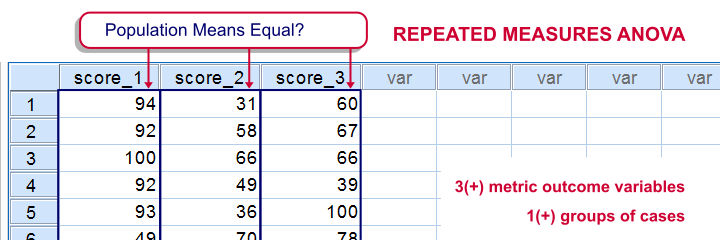SPSS repeated measures ANOVA tests if the means of 3 or more metric variables are all equal in some population. If this is true and we inspect a sample from our population, the sample means may differ a little bit. Large sample differences, however, are unlikely; these suggest that the population means weren't equal after all.
The simplest repeated measures ANOVA involves 3 outcome variables, all measured on 1 group of cases (often people). Whatever distinguishes these variables (sometimes just the time of measurement) is the within-subjects factor.

## Repeated Measures ANOVA Example

A marketeer wants to launch a new commercial and has four concept versions. She shows the four concepts to 40 participants and asks them to rate each one of them on a 10-point scale, resulting in commercial_ratings.sav.Although such ratings are strictly ordinal variables, we'll treat them as metric variables under the assumption of equal intervals. Part of these data are shown below.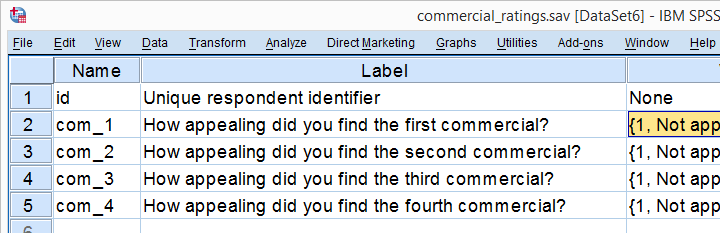The research question is: which commercial has the highest mean rating? We'll first just inspect the mean ratings in our sample. We'll then try and generalize this sample outcome to our population by testing the null hypothesis that the 4 population mean scores are all equal. We'll reject this if our sample means are very different. Reversely, our sample means being slightly different is a normal sample outcome if population means are all similar.

## 2. Assumptions Repeated Measures ANOVA

Running a statistical test doesn't always make sense; results reflect reality only insofar as relevant assumptions are met. For a (single factor) repeated measures ANOVA these are

1. Independent observations (or, more precisely, independent and identically distributed variables). This is often -not always- satisfied by each case in SPSS representing a different person or other statistical unit.
2. The test variables follow a multivariate normal distribution in the population. However, this assumption is not needed if the sample size >= 25.
3. Sphericity. This means that the population variances of all possible difference scores (com_1 - com_2, com_1 - com_3 and so on) are equal. Sphericity is tested with Mauchly’s test which is always included in SPSS’ repeated measures ANOVA output so we'll get to that later.

## 3. Quick Data Check

Before jumping blindly into statistical tests, let's first get a rough idea of what the data look like. Do the frequency distributions look plausible? Are there any system missing values or user missing values that we need to define? For quickly answering such questions, we'll open the data and run histograms with the syntax below.

*Run histograms for 4 variables without frequency tables.

frequencies com_1 to com_4
/format notable
/histogram.

## Result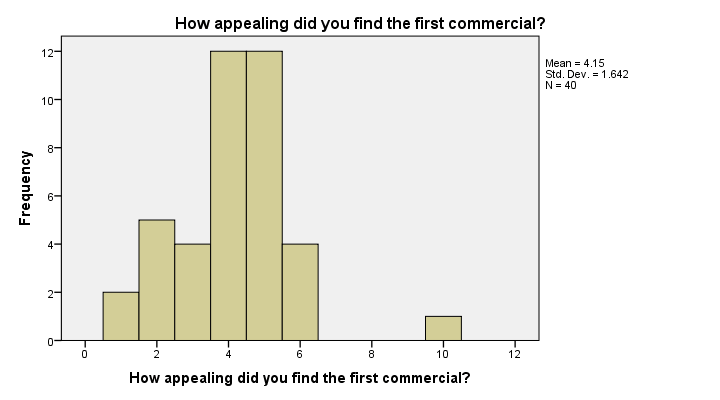First off, our histograms look plausible and don't show any weird patterns or extreme values. There's no need to exclude any cases or define user missing values.
Second, since n = 40 for all variables, we don't have any system missing values. In this case, we can proceed with confidence.

## 4. Run SPSS Repeated Measures ANOVAWe may freely choose a name for our within-subjects factor. We went with “commercial” because it's the commercial that differs between the four ratings made by each respondent.We may also choose a name for our measure: whatever each of the four variables is supposed to reflect. In this case we simply chose “rating”.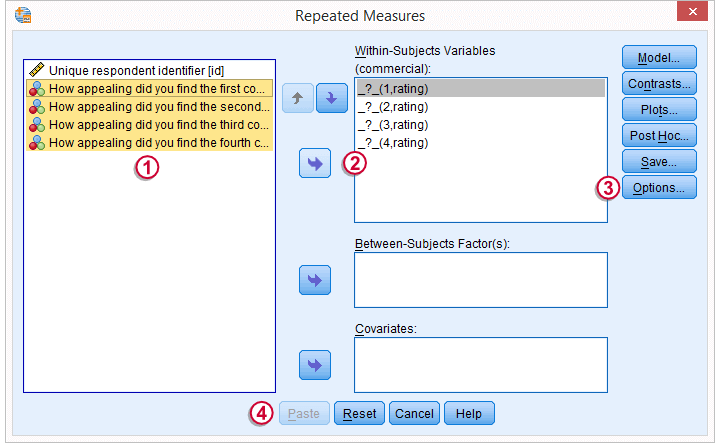We now select all four variables and move them to the Within-subjects variables box withthe arrow to the right.Under Options we'll select Descriptive statistics.Clicking results in the syntax below.

*Basic Repeated-Measures ANOVA Syntax.

GLM com_1 com_2 com_3 com_4
/WSFACTOR=commercial 4 Polynomial
/MEASURE=rating
/METHOD=SSTYPE(3)
/PRINT=DESCRIPTIVE
/CRITERIA=ALPHA(.05)
/WSDESIGN=commercial.

## 5. Repeated Measures ANOVA Output - Descriptives

First off, we take a look at the Descriptive Statistics table shown below. Commercial 4 was rated best (m = 6.25). Commercial 1 was rated worst (m = 4.15). Given our 10-point scale, these are large differences.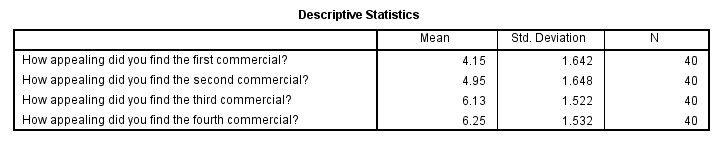## Repeated Measures ANOVA Output - Mauchly’s Test

We now turn to Mauchly's test for the sphericity assumption. As a rule of thumb, sphericity is assumed if Sig. > 0.05. For our data, Sig. = 0.54 so sphericity is no issue here.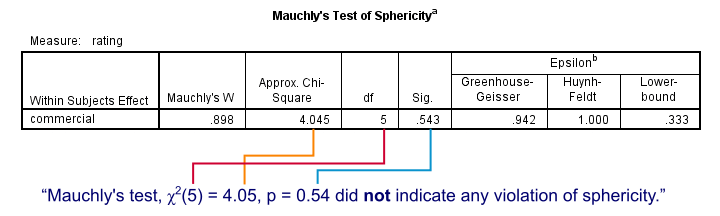The amount of sphericity is estimated by epsilon (the Greek letter ‘e’ and written as ε). There are different ways for estimating it, including the Greenhouse-Geisser, Huynh-Feldt and lower bound methods. If sphericity is violated, these are used to correct the within-subjects tests as we'll see below.If sphericity is very badly violated, we may report the Multivariate Tests table or abandon repeated measures ANOVA altogether in favor of a Friedman test.

## Repeated Measures ANOVA Output - Within-Subjects Effects

Since our data seem spherical, we'll ignore the Greenhouse-Geisser, Huynh-Feldt and lower bound results in the table below. We'll simply interpret the uncorrected results denoted as “Sphericity Assumed”.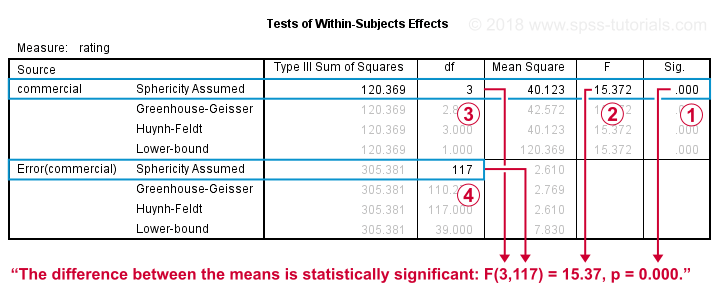The Tests of Within-Subjects Effects is our core output. Because we've just one factor (which commercial was rated), it's pretty simple.Our p-value, Sig. = .000. So if the means are perfectly equal in the population, there's a 0% chance of finding the differences between the means that we observe in the sample. We therefore reject the null hypothesis of equal means.The F-value is not really interesting but we'll report it anyway. The same goes forthe effect degrees of freedom (df1) andthe error degrees of freedom (df2).

## 6. Reporting the Measures ANOVA Result

When reporting a basic repeated-measures ANOVA, we usually report

• the descriptive statistics table
• the outcome of Mauchly's test and
• the outcome of the within-subjects tests.

When reporting corrected results (Greenhouse-Geisser, Huynh-Feldt or lower bound), indicate which of these corrections you used. We'll cover this in SPSS Repeated Measures ANOVA - Example 2.
Finally, the main F-test is reported as “The four commercials were not rated equally,
F(3,117) = 15.4, p = .000.”

Thank you for reading!

# Tell us what you think!

*Required field. Your comment will show up after approval from a moderator.

# THIS TUTORIAL HAS 49 COMMENTS:

•### By David on April 11th, 2020

I've just found your website and, even though it wasn't specifically what I was looking for, I am happily surprised because all the topics are really well explained . The tutorials have also helped me to understand some statistical concepts that I was struggling with. Thanks

•### By anis on June 2nd, 2020

I would like to ask why your final reporting “The four commercials were not rated equally,
F(3,117) = 15.4, p = .000.”
is not answering the research question "which commercial has the highest mean rating"?

•### By Ruben Geert van den Berg on June 3rd, 2020

That question is answered here.

Like we indicated, you should include these descriptives in your final report as well.

Hope that helps!

SPSS tutorials

•### By Mara on January 21st, 2021

This just saved my sanity! Thank you so much. I've spent hours faffing about running all sorts of tests, found this, and did it all in under an hour. Thank you again!!

•### By Alka Gore on February 22nd, 2021

Very nicely explained. Clearly understandable.
Thank you for the tutorials.